# NCERT Solutions For Class 12 Maths Chapter 12 Exercise 12.2

Go back to  'Linear Programming'

## Chapter 12 Ex.12.2 Question 1

Reshma wishes to mix two types of food $$P$$ and $$Q$$ in such a way that the vitamin contents of the mixture contain at least $$8$$ units of vitamin $$A$$ and $$11$$ units of vitamin $$B$$. Food $$P$$ costs ₹ $$60$$/kg and Food Q costs ₹ $$80$$/kg. Food $$P$$ contains $$3$$ units/kg of vitamin $$A$$ and $$5$$ units/kg of vitamin $$B$$ while food $$Q$$ contains $$4$$units/kg of vitamin $$A$$ and $$2$$ units/kg of vitamin $$B$$. Determine the minimum cost of the mixture.

### Solution

Let the mixture contain $$x$$ kg of food $$P$$ and $$y$$ kg of food $$Q$$.

Therefore, $$x \ge 0$$ and $$y \ge 0$$.

The given information can be shown in a table as follows:

 Vitamin A (units/kg) Vitamin B (units/kg) Cost (₹/kg) Food P $$3$$ $$5$$ $$60$$ Food Q $$4$$ $$2$$ $$80$$ Requirement (units/kg) $$8$$ $$11$$

The mixture must contain at least $$8$$ units of Vitamin $$A$$ and $$11$$ units of Vitamin $$B$$.

Thus, the constraints are

\begin{align}3x + 4y &\ge 8\\5x + 2y &\ge 11\end{align}

Total cost, $$Z$$, of purchasing food is

$Z = 60x + 80y$

The mathematical formulation of the given problem is

Minimize

$Z = 60x + 80y\;\;\;\;\;\;\;\;\;\;\; \ldots \left( 1 \right)$

Subject to the constraints,

\begin{align}&3x + 4y \ge 8 \qquad \qquad \ldots \left( 2 \right)\\&5x + 2y \ge 11\qquad \quad \; \;\ldots \left( 3 \right)\\&x,y \ge 0\qquad \qquad\quad \;\; \ldots \left( 4 \right)\end{align}

The feasible region determined by the system of constraints is given by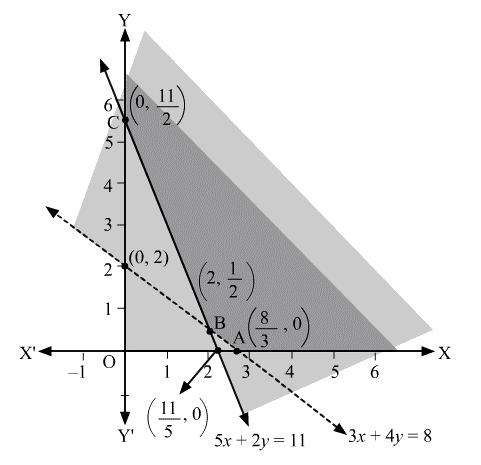Since, the feasible region is unbounded.

The corner points of the feasible region are $$A\left( {\frac{8}{3},0} \right),B\left( {2,\frac{1}{2}} \right)$$ and $$C\left( {0,\frac{{11}}{2}} \right)$$.

The values of $$Z$$ at these corner points are as follows:

 Corner point $$Z = 60x + 80y$$ $${\rm{A}}\left( {\frac{8}{3},0} \right)$$ $$160$$ $$\left. {} \right\} \to$$Minimum $${\rm{B}}\left( {2,\frac{1}{2}} \right)$$ $$160$$ $${\rm{C}}\left( {0,\frac{{11}}{2}} \right)$$ $$440$$

As the feasible region is unbounded, therefore, $$160$$ may or may not be the minimum value of $$Z$$.

For this, we graph the inequality, $$60x + 80y < 160$$ or $$3x + 4y < 8$$ and check whether the resulting half plane has points in common with the feasible region or not.

Since, the feasible region has no common point with $$3x + 4y < 8$$

Thus, the minimum cost of the mixture will be ₹ $$160$$ at the line segment joining the points $$\left( {\frac{8}{3},0} \right)$$ and $$\left( {2,\frac{1}{2}} \right)$$.

## Chapter 12 Ex.12.2 Question 2

One kind of cake requires $$200$$ g flour and $$25$$ g of fat, and another kind of cake requires $$100$$ g of flour and $$50$$ g of fat. Find the maximum number of cakes which can be made from $$5$$ kg of flour and $$1$$ kg of fat assuming that there is no shortage of the other ingredients used in making the cakes.

### Solution

Let there be $$x$$ cakes of first kind and $$y$$ cakes of second kind.

Therefore, $$x \ge 0$$ and $$y \ge 0$$.

The given information can be shown in a table as follows:

 Flour (g) Fat (g) Cakes of first kind, x 200 25 Cakes of second kind, y 100 50 Availability 5000 1000

Hence,

\begin{align}&200x + 100y \le 5000\\&\Rightarrow \; 2x + y \le 50\end{align}

And

\begin{align}&25x + 50y \le 1000\\&\Rightarrow \;x + 2y \le 40\end{align}

Total number of cakes, $$Z$$, that can be made are,

$Z = x + y$

The mathematical formulation of the given problem is

Maximize,

$Z = x + y\;\;\;\;\;\;\;\;\;\; \ldots \left( 1 \right)$

Subject to the constraints,

\begin{align}&2x + y \le 50 \qquad \dots \left( 2 \right)\\&x + 2y \le 40\qquad \dots \left( 3 \right)\\&x,y \ge 0\qquad \quad \;\; \dots \left( 4 \right)\end{align}

The feasible region determined by the system of constraints is given by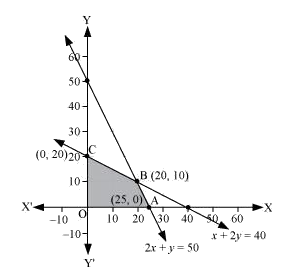The corner points are $$A\left( {25,0} \right),B\left( {20,10} \right),O\left( {0,0} \right)$$ and $$C\left( {0,20} \right)$$.

The value of $$Z$$ at these corner points are as follows:

 Corner point $$Z = x + y$$ $${\rm{A}}\left( {25,0} \right)$$ 25 $${\rm{B}}\left( {20,10} \right)$$ 30 $$\to$$Maximum $${\rm{O}}\left( {0,0} \right)$$ 0 $${\rm{C}}\left( {0,20} \right)$$ 20

Thus, the maximum number of cakes that can be made are $$30$$ ($$20$$ of one kind and $$10$$ of the other kind).

## Chapter 12 Ex.12.2 Question 3

A factory makes tennis rackets and cricket bats. A tennis racket takes $$1.5$$ hours of machine time and $$3$$ hours of craftsman’s time in its making while a cricket bat takes $$3$$ hour of machine time and $$1$$ hour of craftsman’s time. In a day, the factory has the availability of not more than $$42$$ hours of machine time and $$24$$ hours of craftsman’s time.

(i) What number of rackets and bats must be made if the factory is to work at full capacity?

(ii) If the profit on a racket and on a bat is ₹$$20$$ and ₹$$10$$ respectively, find the maximum profit of the factory when it works at full capacity.

### Solution

(i) Let the number of rackets and the number of bats to be made are $$x$$ and $$y$$, respectively.

The machine time is not available for more than $$42$$ hours.

Therefore,

$1.5x + 3y \le 42\;\;\;\;\;\;\;\;\;\; \ldots \left( 1 \right)$

The craftsman’s time is not available for more than $$24$$ hours.

$3x + y \le 24\;\;\;\;\;\;\;\;\; \ldots \left( 2 \right)$

The factory is to work at full capacity.

Therefore,

\begin{align}1.5x + 3y = 42\\3x + y = 24\end{align}

On solving these equations, we obtain

$$x = 4$$ and $$y = 12$$

Thus, $$4$$ rackets and $$12$$ bats must be made.

(ii) The given information can be shown in a table as follows:

 Tennis Racket Cricket Bat Availability Machine Time (h) $$1.5$$ $$3$$ $$42$$ Craftsman’s Time (h) $$3$$ $$1$$ $$24$$

Hence,

\begin{align}&1.5x + 3y \le 42\\&3x + y \le 24\\&x,\;y \ge 0\end{align}

The profit on a racket is ₹ $$20$$ and on bat is ₹ $$10$$

Maximize

$Z = 20x + 10y\;\;\;\;\;\;\;\;\; \ldots \left( 1 \right)$

Subject to the constraints,

\begin{align}&1.5x + 3y \le 42\;\;\;\;\;\;\;\;\; \ldots \left( 2 \right)\\&3x + y \le 24\;\;\;\;\;\;\;\;\;\;\;\;\; \ldots \left( 3 \right)\\&x,\;y \ge 0\;\;\;\;\;\;\;\;\;\;\;\;\;\;\;\;\;\; \ldots \left( 4 \right)\end{align}

The feasible region determined by the system of constraints is given by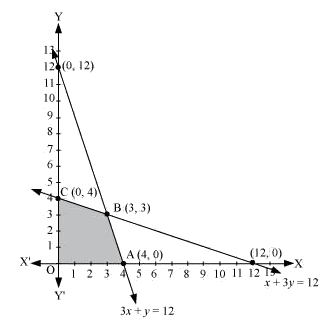The corner points are $$A\left( {8,0} \right),B\left( {4,12} \right),C\left( {0,14} \right)$$ and $$O\left( {0,0} \right)$$.

The values of $$Z$$ at these corner points are as follows:

 Corner point $$Z = 20x + 10y$$ $${\rm{A}}\left( {8,0} \right)$$ $$160$$ $${\rm{B}}\left( {4,12} \right)$$ $$200$$ $$\to$$Maximum $${\rm{C}}\left( {0,14} \right)$$ $$140$$ $${\rm{O}}\left( {0,0} \right)$$ $$0$$

Thus, the maximum profit of the factory when it works to its full capacity is ₹ $$200.$$

## Chapter 12 Ex.12.2 Question 4

A manufacturer produces nuts and bolts. It takes $$1$$ hour of work on machine $$A$$ and $$3$$ hours on machine $$B$$ to produce a package of nuts. It takes $$3$$ hours on machine $$A$$ and $$1$$ hour on machine $$B$$ to produce a package of bolts. He earns a profit, of ₹ $$17.50$$ per package on nuts and ₹ $$7.00$$ per package on bolts. How many packages of each should be produced each day so as to maximize his profit, if he operates his machines for at the most $$12$$ hours a day?

### Solution

Let the manufacturer produce $$x$$ packages of nuts and $$y$$ packages of bolts.

Therefore, $$x \ge 0$$ and $$y \ge 0$$

The given information can be shown in a table as follows:

 Nuts Bolts Availability Machine A (h) $$1$$ $$3$$ $$12$$ Machine B (h) $$3$$ $$1$$ $$12$$

The profit on a package of nuts is ₹ $$17.50$$ and on a package of bolts is ₹ $$7.$$

Therefore, the constraints are

\begin{align}x + 3y \le 12\\3x + y \le 12\end{align}

Total profit,

$Z = 17.5x + 7y$

The mathematical formulation of the given problem is

Maximize

$Z = 17.5x + 7y\;\;\;\;\;\;\;\;\;\; \ldots \left( 1 \right)$

Subject to the constraints,

\begin{align}&x + 3y \le 12\;\;\;\;\;\;\;\;\;\; \ldots \left( 2 \right)\\&3x + y \le 12\;\;\;\;\;\;\;\;\;\; \ldots \left( 3 \right)\\&x,\;y \ge 0\;\;\;\;\;\;\;\;\;\;\;\;\;\;\; \ldots \left( 4 \right)\end{align}

The feasible region determined by the system of constraints is given byThe corner points are $$O\left( {0,0} \right),A\left( {4,0} \right),B\left( {3,3} \right)$$ and $$C\left( {0,4} \right)$$.

The values of $$Z$$ at these corner points are as follows:

 Corner point $$Z = 17.5x + 7y$$ $${\rm{O}}\left( {0,0} \right)$$ $$0$$ $${\rm{A}}\left( {4,0} \right)$$ $$70$$ $${\rm{B}}\left( {3,3} \right)$$ $$73.5$$ $$\to$$Maximum $${\rm{C}}\left( {0,4} \right)$$ $$28$$

The maximum value of $$Z$$ is ₹ $$73.50$$ at $$B\left( {3,3} \right)$$.

## Chapter 12 Ex.12.2 Question 5

A factory manufactures two types of screws, $$A$$ and $$B$$. Each type of screw requires the use of two machines, an automatic and a hand operated. It takes $$4$$minutes on the automatic and $$6$$ minutes on hand operated machines to manufacture a package of screws A, while it takes $$6$$ minutes on automatic and $$3$$ minutes on the hand operated machines to manufacture a package of screws $$B$$. Each machine is available for at the most 4 hours on any day. The manufacturer can sell a package of screws A at a profit of ₹ $$7$$ and screws $$B$$ at a prof it of ₹ $$10$$. Assuming that he can sell all the screws he manufactures, how many packages of each type should the factory owner produce in a day in order to maximize his profit? Determine the maximum profit.

### Solution

Let the factory manufacture $$x$$ screws of type $$A$$ and $$y$$ screws of type $$B$$ on each day.

Therefore, $$x \ge 0$$ and $$y \ge 0$$

The given information can be shown in a table as follows:

 Screw A Screw B Availability Automatic Machine (min.) $$4$$ $$6$$ $$4 \times 60 = 240$$ Hand Operated Machine (min.) $$6$$ $$3$$ $$4 \times 60 = 240$$

The profit on a package of screws $$A$$ is ₹ $$7$$ and on the package of screws $$B$$ is ₹ $$10.$$

Therefore, the constraints are

\begin{align}4x + 6y \le 240\\6x + 3y \le 240\end{align}

Total profit,

$Z = 7x + 10y$

The mathematical formulation of the given problem is

Maximize

$Z = 7x + 10y\;\;\;\;\;\;\;\;\;\; \ldots \left( 1 \right)$

Subject to the constraints,

\begin{align}4x + 6y \le 240\;\;\;\;\;\;\;\;\;\; \ldots \left( 2 \right)\\6x + 3y \le 240\;\;\;\;\;\;\;\;\;\; \ldots \left( 3 \right)\\x,\;y \ge 0\;\;\;\;\;\;\;\;\;\;\;\;\;\;\;\;\;\; \ldots \left( 4 \right)\end{align}

The feasible region determined by the system of constraints is given by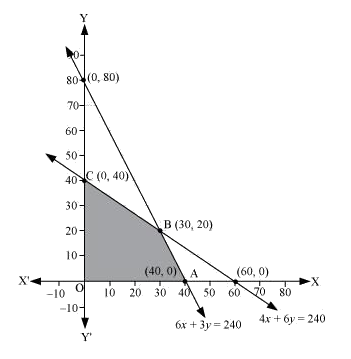The corner points are $$A\left( {40,0} \right),B\left( {30,20} \right)$$ and $$C\left( {0,40} \right)$$.

The values of $$Z$$ at these corner points are as follows:

 Corner point $$Z = 7x + 10y$$ $${\text{A}}\left( {40,0} \right)$$ $$280$$ $${\rm{B}}\left( {30,20} \right)$$ $$410$$ $$\to$$Maximum $${\rm{C}}\left( {0,40} \right)$$ $$400$$

The maximum value of $$Z$$ is $$410$$ at $$B\left( {30,20} \right)$$.

## Chapter 12 Ex.12.2 Question 6

A cottage industry manufactures pedestal lamps and wooden shades, each requiring the use of a grinding /cutting machine and a sprayer. It takes $$2$$ hours on grinding /cutting machine and $$3$$ hours on the sprayer to manufacture a pedestal lamp. It takes $$1$$ hour on the grinding /cutting machine and $$2$$ hours on the sprayer to manufacture a shade. On any day, the sprayer is available for at the most $$20$$ hours and the grinding/cutting machine for at the most $$12$$ hours. The profit from the sale of a lamp is ₹ $$5$$ and that from a shade is ₹ $$3$$. Assuming that the manufacturer can sell all the lamps and shades that he produces, how should he schedule his daily production in order to maximize his profit?

### Solution

Let the cottage industry manufacture $$x$$ pedestal lamps and $$y$$ wooden shades.

Therefore, $$x \ge 0$$ and $$y \ge 0$$

The given information can be shown in a table as follows:

 Lamps Shades Availability Grinding/Cutting Machine (h) $$2$$ $$1$$ $$12$$ Sprayer (h) $$3$$ $$2$$ $$20$$

The profit on a lamp is ₹ $$5$$ and on the shades is ₹ $$3.$$

Therefore, the constraints are

\begin{align}2x + y \le 12\\3x + 2y \le 20\end{align}

Total profit,

$Z = 5x + 3y$

The mathematical formulation of the given problem is

Maximize

$Z = 5x + 3y\;\;\;\;\;\;\;\;\;\; \ldots \left( 1 \right)$

Subject to the constraints,

\begin{align}&2x + y \le 12\;\;\;\;\;\;\;\;\;\; \ldots \left( 2 \right)\\&3x + 2y \le 20\;\;\;\;\;\;\;\; \ldots \left( 3 \right)\\&x,\;y \ge 0\;\;\;\;\;\;\;\;\;\;\;\;\;\;\; \ldots \left( 4 \right)\end{align}

The feasible region determined by the system of constraints is given by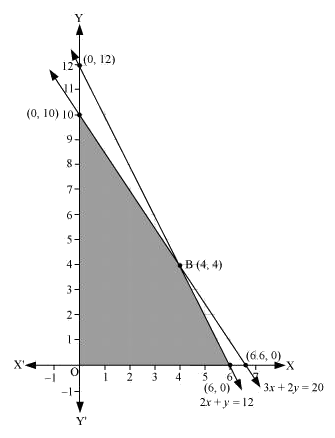The corner points are $$A\left( {6,0} \right),B\left( {4,4} \right)$$ and $$C\left( {0,10} \right)$$.

The values of $$Z$$ at these corner points are as follows:

 Corner point $$Z = 5x + 3y$$ $${\rm{A}}\left( {6,0} \right)$$ $$30$$ $${\rm{B}}\left( {4,4} \right)$$ $$32$$ $$\to {\rm{Maximum}}$$ $${\rm{C}}\left( {0,10} \right)$$ $$30$$

The maximum value of $$Z$$ is $$32$$ at $$B\left( {4,4} \right)$$.

Therefore, the manufacturer should produce $$4$$ pedestal lamps and $$4$$ wooden shades to maximize his profits.

## Chapter 12 Ex.12.2 Question 7

A company manufactures two types of novelty Souvenirs made of plywood. Souvenirs of type $$A$$ require $$5$$ minutes each for cutting and $$10$$ minutes each for assembling. Souvenirs of type $$B$$ require $$8$$ minutes each for cutting and $$8$$ minutes each for assembling. There are $$3$$ hours $$20$$ minutes available for cutting and $$4$$ hours of assembling. The profit is ₹ $$5$$ each for type $$A$$ and ₹ $$6$$ each for type $$B$$ Souvenirs. How many Souvenirs of each type should the company manufacture in order to maximize the profit?

### Solution

Let the company manufacture $$x$$ Souvenirs of type $$A$$ and $$y$$ Souvenirs of type $$B.$$

Therefore, $$x \ge 0$$ and $$y \ge 0$$.

The given information can be shown in a table as follows:

 Type A Type B Availability Cutting (min.) $$5$$ $$8$$ $$3 \times 60 + 20 = 200$$ Assembling (min.) $$10$$ $$8$$ $$4 \times 60 = 240$$

The profit on type $$A$$ Souvenirs is ₹ $$5$$ and type $$B$$ Souvenirs is ₹ $$6$$.

Therefore, the constraints are

$5x + 8y \le 200$

And

\begin{align}10x + 8y \le 240\\ \Rightarrow 5x + 4y \le 120\end{align}

Total profit,

$Z = 5x + 6y$

The mathematical formulation of the given problem is

Maximize

$Z = 5x + 6y\;\;\;\;\;\;\;\;\;\; \ldots \left( 1 \right)$

Subject to the constraints,

\begin{align}&5x + 8y \le 200\;\;\;\;\;\;\;\;\;\; \ldots \left( 2 \right)\\&5x + 4y \le 120\;\;\;\;\;\;\;\;\;\; \ldots \left( 3 \right)\\&x,\;y \ge 0\;\;\;\;\;\;\;\;\;\;\;\;\;\;\;\;\;\; \ldots \left( 4 \right)\end{align}

The feasible region determined by the system of constraints is given by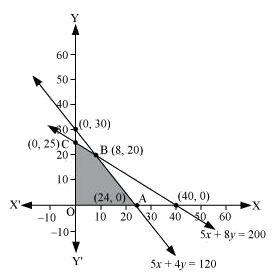The corner points are $$A\left( {24,0} \right),B\left( {8,20} \right)$$ and $$C\left( {0,25} \right)$$.

The values of $$Z$$ at these corner points are as follows:

 Corner point $$Z = 5x + 6y$$ $${\rm{A}}\left( {24,0} \right)$$ $$120$$ $${\rm{B}}\left( {8,20} \right)$$ $$160$$ $$\to$$Maximum $${\rm{C}}\left( {0,25} \right)$$ $$150$$

The maximum value of $$Z$$ is $$160$$ at $$B\left( {8,20} \right)$$.

Hence, $$8$$ Souvenirs of type $$A$$ and $$20$$ Souvenirs of type $$B$$ should be produced each day to get the maximum profit of ₹ $$160.$$

## Chapter 12 Ex.12.2 Question 8

A merchant plans to sell two types of personal computers − a desktop model and a portable model that will cost ₹ $$25000$$ and ₹ $$40000$$ respectively. He estimates that the total monthly demand of computers will not exceed $$250$$ units. Determine the number of units of each type of computers which the merchant should stock to get maximum profit if he does not want to invest more than ₹ $$70$$ lakhs and if h is profit on the desktop model is ₹ $$4500$$ and on portable model is ₹ $$5000$$.

### Solution

Let the merchant stock $$x$$ desktop models and $$y$$ portable models.

Therefore, $$x \ge 0$$ and $$y \ge 0$$

The cost of a desktop model is ₹ $$25000$$ and of a portable model is ₹ $$4000$$. However, the merchant can invest a maximum of ₹ $$70$$ lakhs.

Therefore,

\begin{align}&25000x + 40000y \le 7000000\\&\Rightarrow 5x + 8y \le 1400\end{align}

The monthly demand of computers will not exceed $$250$$ units.

Therefore,

$x + y \le 250$

The profit on a desktop model is ₹ $$4500$$ and the profit on a portable model is ₹ $$5000$$.

Total profit,

$Z = 4500x + 5000y$

Thus, the mathematical formulation of the given problem is

Maximize

$Z = 4500x + 5000y\;\;\;\;\;\;\;\;\;\; \ldots \left( 1 \right)$

Subject to the constraints,

\begin{align}&5x + 8y \le 1400\;\;\;\;\;\;\;\;\;\; \ldots \left( 2 \right)\\&x + y \le 250\;\;\;\;\;\;\;\;\;\;\;\;\;\;\; \ldots \left( 3 \right)\\&x,\;y \ge 0\;\;\;\;\;\;\;\;\;\;\;\;\;\;\;\;\;\;\;\; \ldots \left( 4 \right)\end{align}

The feasible region determined by the system of constraints is given by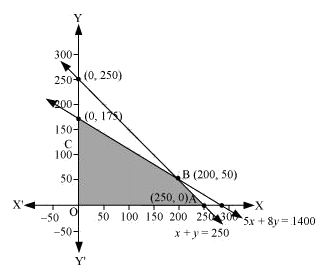The corner points are $$A\left( {250,0} \right),B\left( {200,50} \right)$$ and $$C\left( {0,175} \right)$$.

The values of $$Z$$ at these corner points are as follows:

 Corner point $$Z = 4500x + 5000y$$ $${\rm{A}}\left( {250,0} \right)$$ $$1125000$$ $${\rm{B}}\left( {200,50} \right)$$ $$1150000$$ $$\to$$Maximum ${\rm{C}}\left( {0,175} \right)$ $$875000$$

The maximum value of $$Z$$ is $$1150000$$ at $$B\left( {200,50} \right)$$.

Therefore, the merchant should stock $$200$$ desktop models and $$50$$ portable models to get the maximum profit of ₹ $$1150000.$$

## Chapter 12 Ex.12.2 Question 9

A diet is to contain at least $$80$$ units of vitamin $$A$$ and $$100$$ units of minerals. Two foods $${F_1}$$ and $${F_2}$$ are available. Food $${F_1}$$ costs ₹ $$4$$ per unit food and $${F_2}$$ costs ₹ $$6$$ per unit. One unit of food $${F_1}$$ contains $$3$$ units of vitamin $$A$$ and $$4$$ units of minerals. One unit of food $${F_2}$$ contains $$6$$ units of vitamin $$A$$ and $$3$$ units of minerals. Formulate this as a linear programming problem. Find the minimum cost for diet that consists of mixture of these two foods and also meets the minimal nutritional requirements.

### Solution

Let the diet contain $$x$$ units of food $${F_1}$$ and y units of food $$F_2$$.

Therefore,$$x \ge 0$$ and $$y \ge 0$$

The given information can be shown in a table as follows:

 Vitamin A (units) Mineral (units) Cost per unit (?) Food F1 (x) $$3$$ $$4$$ $$4$$ Food F2 (y) $$6$$ $$3$$ $$6$$ Requirement $$80$$ $$100$$

The cost of food $${F_1}$$ is ₹ $$4/\rm{unit}$$ and of food $${F_2}$$ is ₹ $$6/\rm{unit.}$$

Therefore, the constraints are

\begin{align}3x + 6y \ge 80\\4x + 3y \ge 100\\x,\;y \ge 0\end{align}

Total cost of the diet,

$Z = 4x + 6y$

The mathematical formulation of the given problem is

Minimize

$Z = 4x + 6y\;\;\;\;\;\;\;\;\;\;\; \ldots \left( 1 \right)$

Subject to the constraints,

\begin{align}3x + 6y \ge 80\;\;\;\;\;\;\;\;\;\;\; \ldots \left( 2 \right)\\4x + 3y \ge 100\;\;\;\;\;\;\;\;\; \ldots \left( 3 \right)\\x,\;y \ge 0\;\;\;\;\;\;\;\;\;\;\;\;\;\;\;\;\; \ldots \left( 4 \right)\end{align}

The feasible region determined by the constraints is given by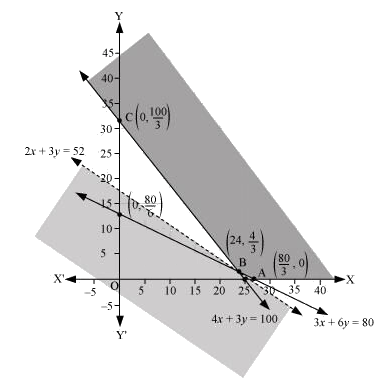Since, the feasible region is unbounded.

The corner points of the feasible region are $$A\left( {\frac{{80}}{3},0} \right),B\left( {24,\frac{4}{3}} \right)$$ and $$C\left( {0,\frac{{100}}{3}} \right)$$.

The values of $$Z$$ at these corner points are as follows:

 Corner point $$Z = 4x + 6y$$ $${\rm{A}}\left( {\frac{{80}}{3},0} \right)$$ $$\frac{{320}}{3} = 106.67$$ $${\rm{B}}\left( {24,\frac{4}{3}} \right)$$ $$104$$ $$\to$$Minimum $${\rm{C}}\left( {0,\frac{{100}}{3}} \right)$$ $$200$$

As the feasible region is unbounded, therefore, $$104$$ may or may not be the minimum value of $$Z.$$

For this, we draw a graph of the inequality, $$4x + 6y < 104$$ or $$2x + 3y < 52$$, and check whether the resulting half plane has points in common with the feasible region or not.

Since, the feasible region has no common point with $$2x + 3y < 52$$.

Therefore, the minimum cost of the mixture will be ₹ 104.

## Chapter 12 Ex.12.2 Question 10

There are two types of fertilizers $${F_1}$$ and $${F_2}$$. $${F_1}$$ consists of 10% nitrogen and 6% phosphoric acid and $${F_2}$$ consists of $$5\%$$ nitrogen and $$10\%$$ phosphoric acid. After testing the soil conditions, a farmer finds that she needs at least $$14\rm{ kg}$$ of nitrogen and $$14\rm{ kg}$$ of phosphoric acid for her crop. If $${F_1}$$ cost ₹ $$6/\rm{kg}$$ and $${F_2}$$ costs ₹ $$5/\rm{kg}$$, determine how much of each type of fertilizer should be used so that nutrient requirements are met at a minimum cost. What is the minimum cost?

### Solution

Let the farmer buy $$x\,\rm{kg}$$ of fertilizer $${F_1}$$ and $$y\,\rm{kg}$$ of fertilizer$${F_2}$$.

Therefore, $$x \ge 0$$ and $$y \ge 0$$

The given information can be shown in a table as follows:

 Nitrogen (%) Phosphoric Acid (%) Cost (?/kg) F1 (x) $$10$$ $$6$$ $$6$$ F2 (y) $$5$$ $$10$$ $$5$$ Requirement (kg) $$14$$ $$14$$

$${F_1}$$ consists of $$10\%$$nitrogen and $${F_2}$$ consists of $$5\%$$ nitrogen. However, the farmer requires at least $$14\,\rm {kg}$$ of nitrogen.

Therefore,

\begin{align}&10\% \;of\;x + 5\% \;of\;y \ge 14\\&\frac{x}{{10}} + \frac{y}{{20}} \ge 14\\&2x + y \ge 280\end{align}

$${F_1}$$ consists of $$6\%$$ phosphoric acid and $${F_2}$$ consists of $$10\%$$ phosphoric acid.

However, the farmer requires at least $$14\rm{ kg}$$ of phosphoric acid.

Therefore,

\begin{align}&6\% \;of\;x + 10\% \;of\;y &\ge 14\\&\frac{{6x}}{{100}} + \frac{{10y}}{{100}} &\ge 14\\&3x + 5y &\ge 700\end{align}

Total cost of fertilizers,

$Z = 6x + 5y$

The mathematical formulation of the given problem is

Minimize

$Z = 6x + 5y\;\;\;\;\;\;\;\;\; \ldots \left( 1 \right)$

Subject to the constraints,

\begin{align}&2x + y \ge 280\;\;\;\;\;\;\;\;\; \ldots \left( 2 \right)\\&3x + 5y \ge 700\;\;\;\;\;\;\;\; \ldots \left( 3 \right)\\&x,\;y \ge 0\;\;\;\;\;\;\;\;\;\;\;\;\;\;\;\; \ldots \left( 4 \right)\end{align}

The feasible region determined by the system of constraints is given by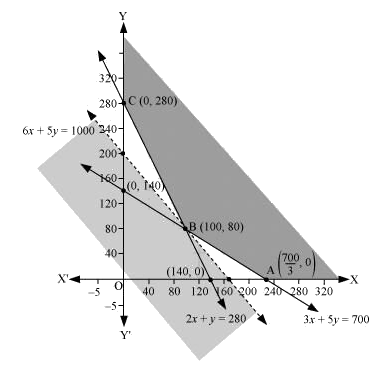Since, the feasible region is unbounded.

The corner points are $$A\left( {\frac{{700}}{3},0} \right),B\left( {100,80} \right)$$ and $$C\left( {0,280} \right)$$.

The values of $$Z$$ at these corner points are as follows:

 Corner point $$Z = 6x + 5y$$ $${\rm{A}}\left( {\frac{{700}}{3},0} \right)$$ $$1400$$ $${\rm{B}}\left( {100,80} \right)$$ $$1000$$ $$\to$$Minimum $${\rm{C}}\left( {0,280} \right)$$ $$1400$$

As the feasible region is unbounded, therefore, $$1000$$ may or may not be the minimum value of $$Z.$$

For this, we draw a graph of the inequality, $$6x + 5y < 1000$$, and check whether the resulting half plane has points in common with the feasible region or not.

Since, the feasible region has no common point with $$6x + 5y < 1000$$.

Thus, $$100\,\rm{ kg}$$ of fertilizer $${F_1}$$ and $$80 \,\rm{kg}$$ of fertilizer $${F_2}$$ should be used to minimize the cost. The minimum cost is ₹ $$1000.$$

## Chapter 12 Ex.12.2 Question 11

The corner points of the feasible region determined by the following system of linear inequalities:

$$2x + y \le 10,\;x + 3y \le 15,\;x,y \ge 0$$ are $$\left( {0,0} \right),\;\left( {5,0} \right),\;\left( {3,4} \right)$$ and $$\left( {0,5} \right)$$. Let $$Z = px + qy,$$ where$$p,\;q > 0$$. Condition on $$p$$ and $$q$$ so that the maximum of $$Z$$ occurs at both$$\left( {3,4} \right)$$ and $$\left( {0,5} \right)$$ is

(A) $$p = q$$

(B) $$p = 2q$$

(C) $$p = 3q$$

(D) $$q = 3p$$

### Solution

The maximum value of $$Z$$ is unique.

It is given that the maximum value of $$Z$$ occurs at two points, $$\left( {3,4} \right)$$ and $$\left( {0,5} \right)$$.

Therefore, Value of Z at $$\left( {3,4} \right) =$$ Value of Z at $$\left( {0,5} \right)$$

\begin{align}&\Rightarrow\; p\left( 3 \right) + q\left( 4 \right) = p\left( 0 \right) + q\left( 5 \right)\\&\Rightarrow \;3p + 4q = 5q\\&\Rightarrow\; q = 3p\end{align}

Thus, the correct option is (D).

Related Sections
Related Sections
Instant doubt clearing with Cuemath Advanced Math Program